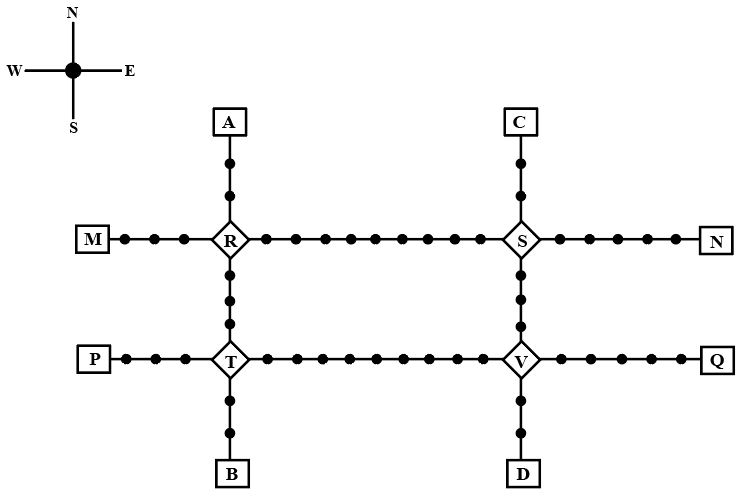### CAT 2022 Question Paper (Slot 1) Question 42

InstructionsGiven above is the schematic map of the metro lines in a city with rectangles denoting terminal stations (e.g. A), diamonds denoting junction stations (e.g. R) and small filled-up circles denoting other stations. Each train runs either in east-west or north-south direction, but not both. All trains stop for 2 minutes at each of the junction stations on the way and for 1 minute at each of the other stations. It takes 2 minutes to reach the next station for trains going in east-west direction and 3 minutes to reach the next station for trains going in northsouth direction. From each terminal station, the first train starts at 6 am; the last trains leave the terminal stations at midnight. Otherwise, during the service hours, there are metro service every 15 minutes in the north-south lines and every 10 minutes in the east-west lines. A train must rest for at least 15 minutes after completing a trip at the terminal station, before it can undertake the next trip in the reverse direction. (All questions are related to this metro service only. Assume that if someone reaches a station exactly at the time a train is supposed to leave, (s)he can catch that train.)

Question 42

# Haripriya is expected to reach station S late. What is the latest time by which she must be ready to board at station S if she must reach station B before 1 am via station R?

Solution

Travelling time between S and R = $$\left(10\times2\right)+\left(9\times1\right)=29\$$ minutes

There is a stoppage of 2 minutes at R

Travelling time between R and B = $$\left(7\times3\right)+\left(1\times2\right)+\left(5\times1\right)=28$$ minutes

In the north-south direction, the first train from A arrives at R at time = 6 am +$$\left(3\times3\right)+\left(2\times1\right)$$ = 6:11 am.

Since R is a junction so this train will halt for 2 minutes at R and leave at 6:13.

Every 15 minutes, a train starts from A in the north-south direction.

The last train that leaves A will be at 12:00 am and it will leave R at 12:13 am, so Haripriya must reach R till 12:13 am.

Travelling time between S and R = $$\left(10\times2\right)+\left(9\times1\right)=29\$$ minutes

So Haripriya must board the train at S by 11:44 pm

In the east-west direction, the first train from N arrives at S at time = 6 am +$$\left(6\times2\right)+\left(5\times1\right)$$ = 6:17 am.

Since S is a junction so this train will halt for 2 minutes at S and leave at 6:19.

Every 10 minutes, a train starts from N in the east-west direction.

Therefore, Haripriya should board the train which leaves S at 11:39.